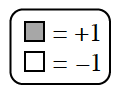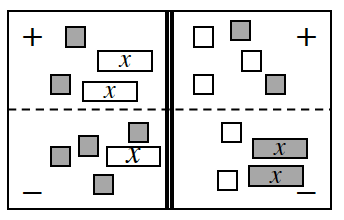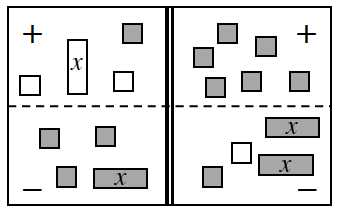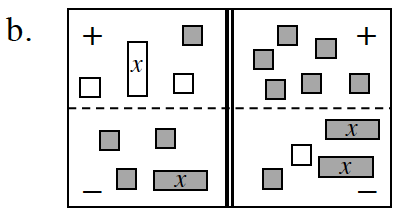### Home > INT1 > Chapter A > Lesson A.1.8 > ProblemA-82

A-82.Solve the equation represented on each Equation Mats below. Record your work. Check your answer.

1.Tackle the Equation Mat corner by corner:top left, bottom left, top right, bottom right.
Remember, the problem does not ask you to simplify.

$\left(−2x + 2\right) − \left(−x + 4\right) =$ ?

1.Use the Equation Mat below to solve the equation.
Click the link at the right to view the full eTool version: A-82a HW eTool.Use the Equation Mat below to solve the equation.
Click the link at the right to view the full eTool version: A-82b HW eTool.